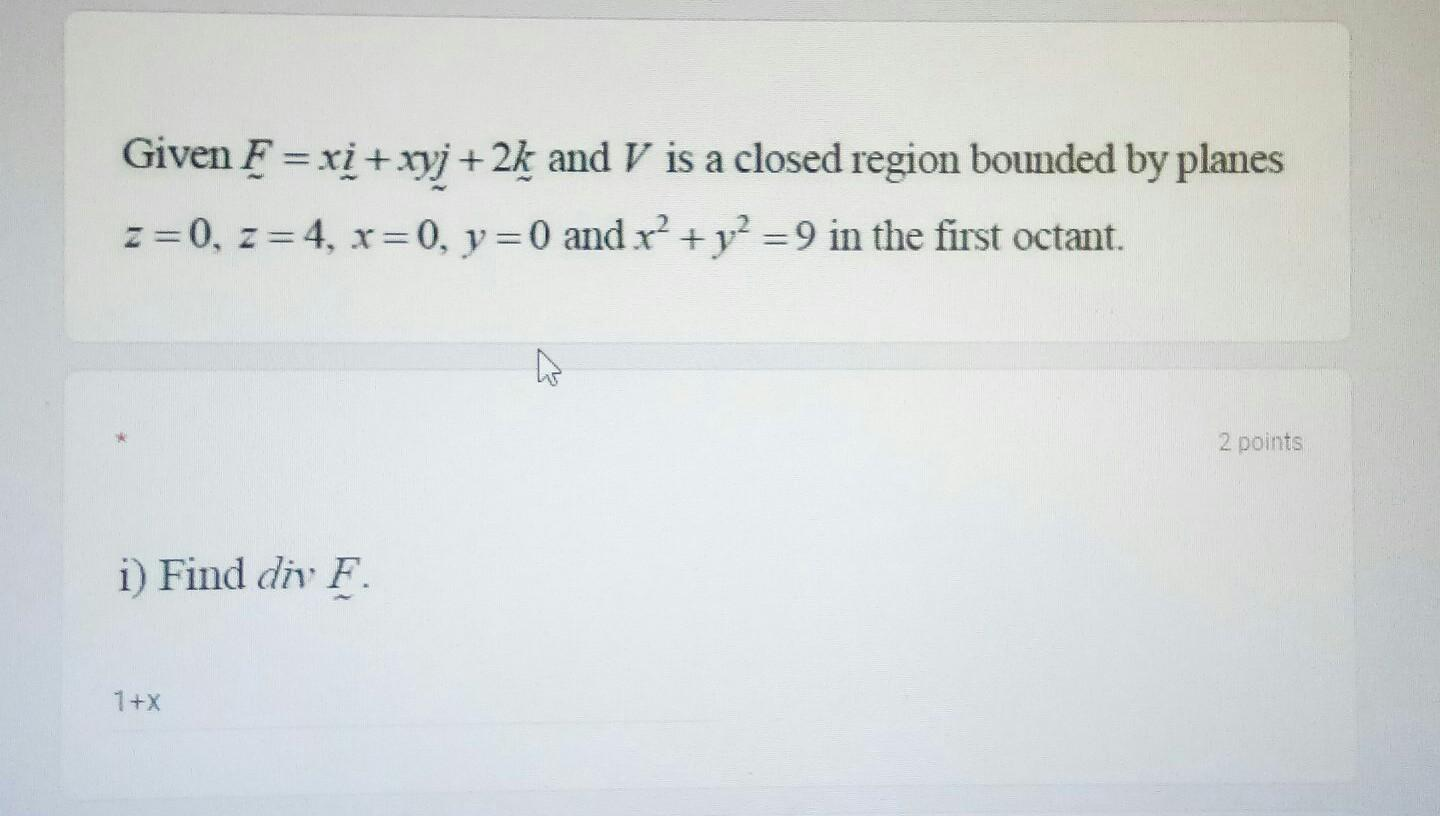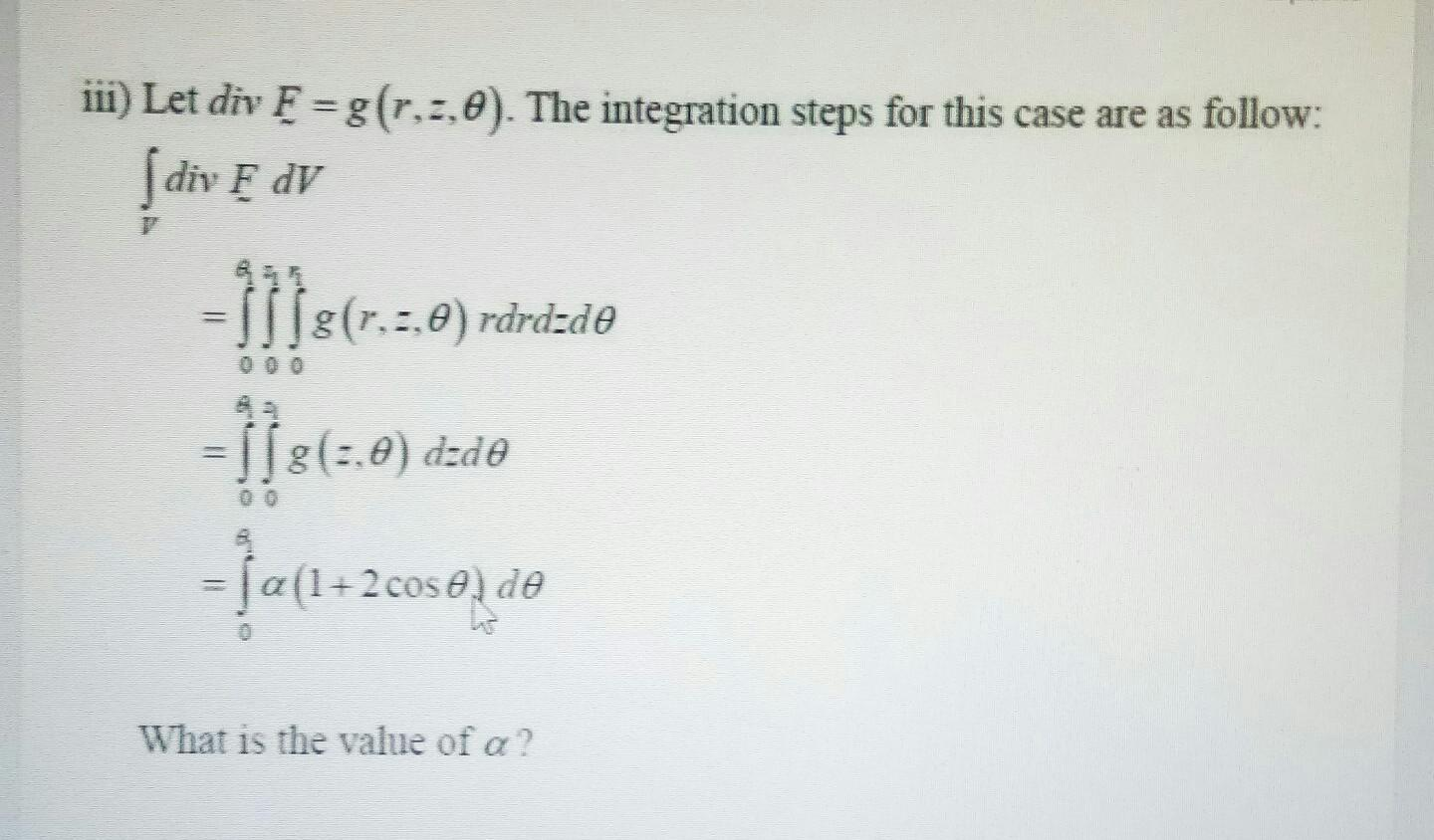### Create an Account

Already have account?

### Forgot Your Password ?

Home / Questions / Given F = xi + xyj +2k and V is a closed region bounded by planes z=0, z =4, x=0, y = 0 an...

# Given F = xi + xyj +2k and V is a closed region bounded by planes z=0, z =4, x=0, y = 0 and r² + y2 =9 in the first octant. 2 points i) Find div F. 1+X iii) Let div F =g(r.2.0). The integration steps

Given F = xi + xyj +2k and V is a closed region bounded by planes z=0, z =4, x=0, y = 0 and r² + y2 =9 in the first octant. 2 points i) Find div F. 1+X
iii) Let div F =g(r.2.0). The integration steps for this case are as follow: diy F DV 1118(7.3.0 ) rdrd:de f}1= - ļa(1+2coso de 55:(:,0) dzde What is the value of a?Apr 14 2021 View more View Less

#### Answer (Solved)Subscribe To Get Solution